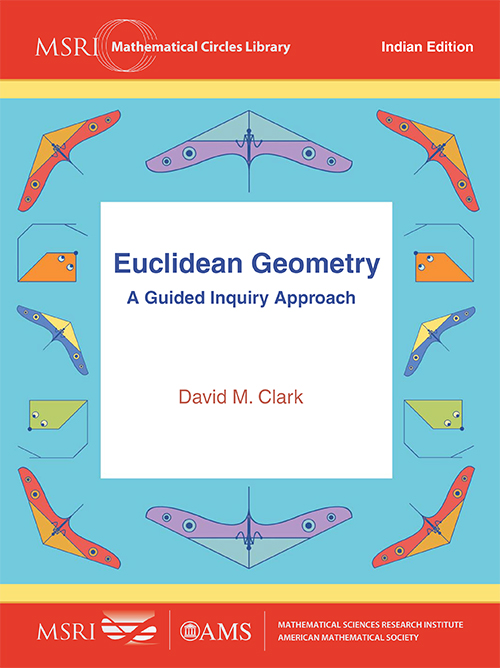## Euclidean Geometry: A Guided Inquiry Approach

#### Price: 780.00

Geometry has been an essential element in the study of mathematics since antiquity. Traditionally, we have also learned formal reasoning by studying Euclidean geometry. In this book, David Clark develops a modern axiomatic approach to this ancient subject, both in content and presentation. Mathematically, Clark has chosen a new set of axioms that draw on a modern understanding of set theory and logic, the real number continuum and measure theory, none of which were available in Euclid’s time. The result is a development of the standard content of Euclidean geometry with the mathematical precision of Hilbert’s foundations of geometry. In particular, the book covers all the topics listed in the Common Core State Standards for high school synthetic geometry. The presentation uses a guided inquiry, active learning pedagogy. Students benefit from the axiomatic development because they themselves solve the problems and prove the theorems with the instructor serving as a guide and mentor. Students are thereby empowered with the knowledge that they can solve problems on their own without reference to authority. This book, written for an undergraduate axiomatic geometry course, is particularly well suited for future secondary school teachers.

#### Contributors (Author(s), Editor(s), Translator(s), Illustrator(s) etc.)

David M Clark is Chair of the Mathematics Department at the State University of New York, New Paltz, USA.

Acknowledgements
Preface
Chapter 1. Congruent Figures
Chapter 2. Axioms, Theorems and Proofs
Chapter 3. Area Measure
Chapter 4. Angle Measure
Chapter 5. Similar Figures
Chapter 6. Trigonometric Ratios
Chapter 7. Circle Measure
Chapter 8. Perspective Geometry
Appendix A. The Axioms
Appendix B. Guidelines for the Instructor
Appendix C. Hilbert’s Axioms
Bibliography
Index

`